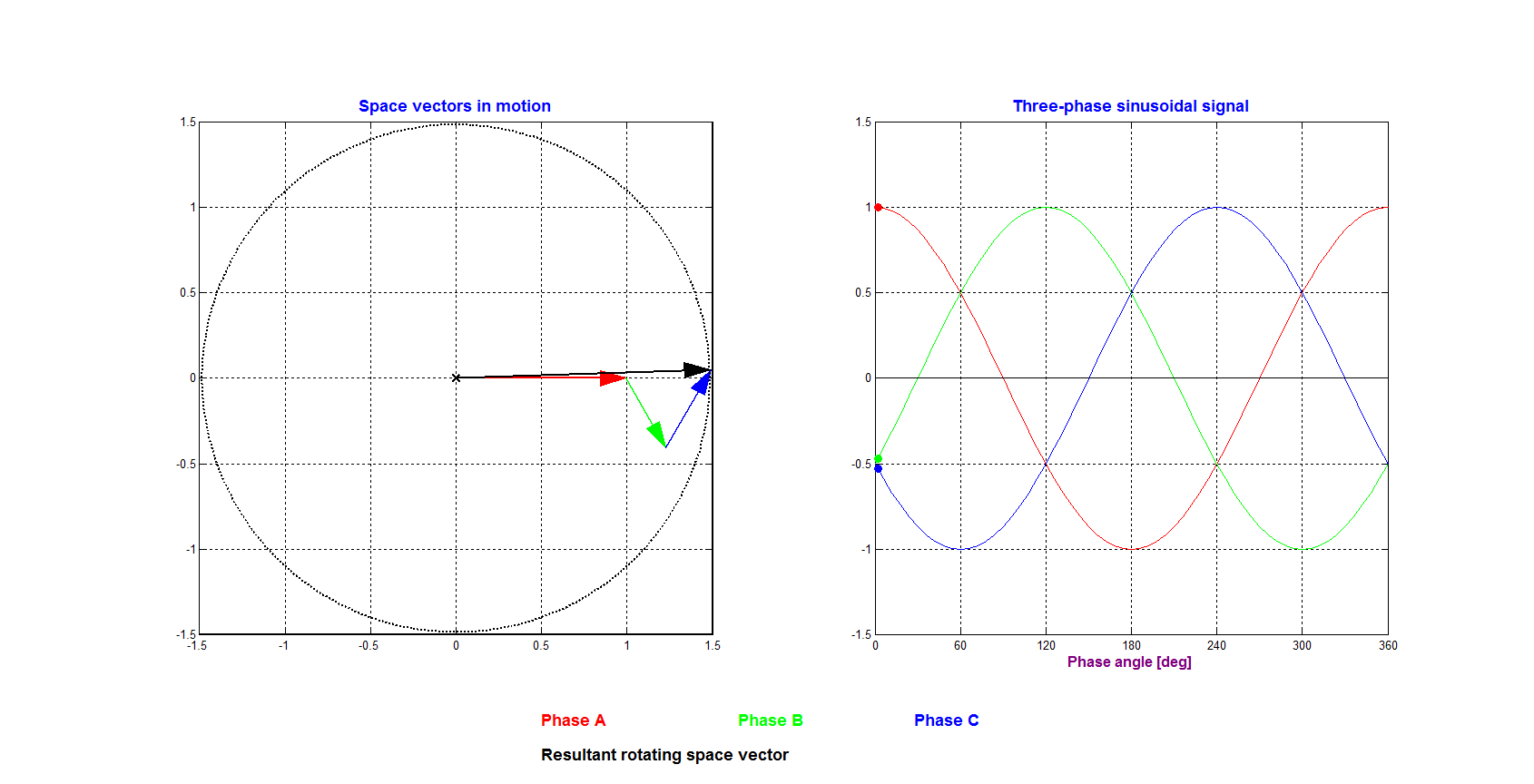﻿ Space vectors of 3-phase sinusoidal signals

SPACE VECTORS

This animation shows the motion of space vectors for the case of a balanced three-phase sinusoidal signal:

fA = cos(ωt), fB = cos(ωt-α), fC = cos(ωt+α)        where α = 2π/3.

The corresponding space vector is obtained from fR = (fA + γ fB + γ2 fC) = 1.5ejωt        where γ = e.